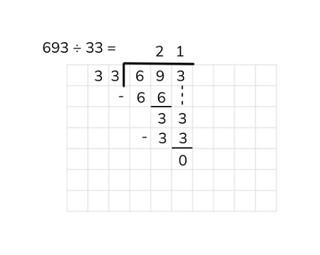Long division with a number to 1,000

# Long division with a number to 1,000

Students learn long division with a number to 1,000.

No account needed.8,000 schools use Gynzy92,000 teachers use Gynzy1,600,000 students use Gynzy

## General

The students learn long division with a number to 1,000 without remainder.

## Standards

CCSS.Math.Content.6.NS.B.2

## Learning objective

Students will be able to use long division to divide a number to 1,000 by a number greater than 10 without remainder.

## Introduction

The students try to subtract repeatedly from 988 to calculate the final answer within 25 seconds.

## Instruction

Explain that with long division the numbers of the problem go next to one another. First you write the divisor on the left. Next you write the dividend on the right and draw a box around it. You will put the answer on top of the box. Show that you first look at the first digit. That is the 5 of 559. You cannot divide this 5 by 13, so you also need the next digit. Then you see how many times 13 can go into 55. Emphasize that you can make a row of the table of 13 to help you with this. In this way you can quickly check and see what the largest number is that you can take away. In this case the largest number is: 4 × 13 = 52. Under 55, write the number 52 and subtract these numbers from one another. You write the answer (3) underneath. You write the 4, from 4 × 13, in the answer on top of the box. 13 does not fit in 3, so you look again to the next digit. Write the 9 of 559 next to the 3. You now have 39. Now you look again to see what the largest number is that you can take away. That is 3 × 13 = 39. Write the 39 under the number 39 and subtract these from each other. You now have 0 left over. You write the 3 from 3 × 13 next to the 4 in your answer. The answer is now 43. Next you can practice the problem 345 ÷ 23 = together with the students. You may remove the cover to show the helping row of problems. Now you can have the students solve the following two problems on their own, using long division.

Check whether the students can divide a number to 1,000 using long division without remainder by asking the following question:
- What steps do you follow to solve the problem 357 ÷ 17 using long division?

## Quiz

The students test their understanding of long division with a number to 1,000 through ten exercises. Some of these exercises involve dividing by a one-digit number as the divisor, and others are with two-digit numbers.

## Closing

Discuss once again the importance of being able to use long division to divide a number to 1,000 without remainder. As a closing activity the students can work in pairs to solve the problems using long division. The students first solve the problems individually and then look at the answer and steps together with their partner. Could either of them have taken larger numbers away?

## Teaching tips

Have students that have difficulty with long division with a number to 1,000 first practice with long division with a number to 100. Have these students first make a helping row, before they start calculating the problem.

## Instruction materials

Scrap paper

### The online teaching platform for interactive whiteboards and displays in schools

• Save time building lessons

• Manage the classroom more efficiently

• Increase student engagement